## Saturday, March 20, 2021

### Flip-Flop (Latch): UNIZOR.COM - Physics4Teens - Electromagnetism - Elect...

Notes to a video lecture on http://www.unizor.com

Implementation of Electronic
Flip Flop (Latch)

In the beginning of computer era one of the first problem was to be able to store information. And the first step was to design a circuit that can store one bit of information, 1 or 0.

A digital flip-flop or latch is such a one bit storage, an electronic device that
(a) has two states (1 and 0);
(b) can be set to one of these two states by a proper impulse;
(c) retains the state after an impulse is gone.

The main component of a design we will discuss in this lecture is a combination of a binary logical operation OR and a unary logical operation NOT, both of which were discussed in previous lectures.

Let's recall the implementation of the logical OR circuit that involves diodes.The implementation of the NOT logical operation that involves triodes was suggested as follows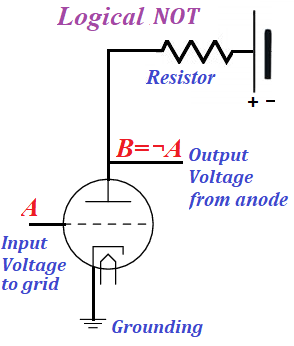Combining them sequentially, we first implement OR and then NOT of the result of OR, thus implementing the binary logical operation NOR as follows
NOT(A OR B) = ¬(A|B)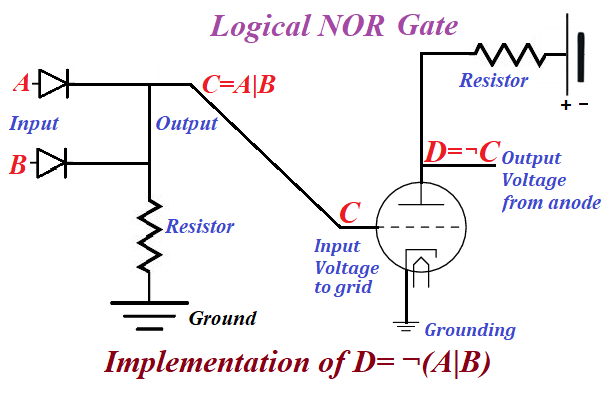The logical NOR operation is denoted by the following symbol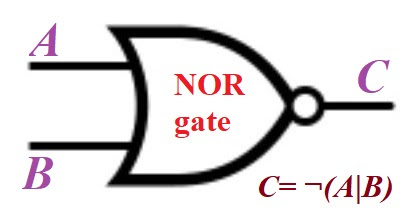The table below denotes the results of NOR operation for all pairs of input values of arguments
¬(0|0) = ¬0 = 1
¬(0|1) = ¬1 = 0
¬(1|0) = ¬1 = 0
¬(1|1) = ¬1 = 0

Using the NOR gate, we will implement a circuit that can serve as a one bit memory cell.

Consider the following schema of a circuit called SR flip-flop or SR latch.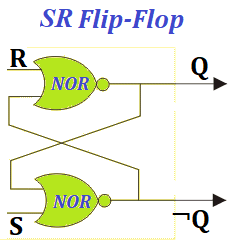Here terminals R (Reset) and S (Set) are controlling terminals, using which we store information.

Information is stored in a pair of terminals Q and its opposite NOT(Q)=¬Q in a way that either Q=1 (and ¬Q=0) or Q=0 (and ¬Q=1).

These two states are stable in a sense that, after we send a positive impulse at terminal S, while R=0, the Q will be equal to 1 irrespective of its prior value, the ¬Q will be equal to 0 irrespective of its prior value, and they both will retain the same values Q=1 and ¬Q=0 after the impulse at S is gone (that is when S=0).

Similarly, after we send a positive impulse at terminal R, while S=0, the Q will be equal to 0 irrespective of its prior value, the ¬Q will be equal to 1 irrespective of its prior value, and they both will retain the same values Q=0 and ¬Q=1 after the impulse at R is gone (that is when R=0).

Let's examine the work of this SR latch in detail.

Set Q=1 and ¬Q=0

Assume, we set the voltage at terminal S to a positive value, keeping terminal R at zero potential. It means in logical sense that S=1 and R=0.
The NOR gate connected to terminal S, irrespective of its second connection to it, will produce a signal 0 as an output. Indeed, the OR operation between S=1 and any other signal produces 1 and NOT(1)=0.

This immediately sets ¬Q=0 and send zero potential to the NOR gate connected to terminal R that has zero potential. So, both inputs to NOR gate connected to R terminal are at zero potential. The logical OR of two zeroes is zero. Subsequent NOT converts the signal to 1 that sets terminal Q=1.
So, raising the potential at terminal S to a positive value, keeping terminal R at zero potential switches terminals Q and ¬Q to, correspondingly, values 1 and 0.

Let's see what happens when the positive impulse at terminal S is gone, so S=0, while terminal R is still at zero potential, terminal Q=1 and ¬Q=0.

The NOR gate connected to terminal S has one input from terminal S=0, while the other input is from terminal Q that was set to 1 on a previous step. The output from this NOR gate will be zero, since the logical OR between 0 and 1 gives 1, and subsequent logical NOT converts it to 0.

This sets ¬Q=0, but this terminal already had this value, so ¬Q does not change and remains at 0.

From there the value ¬Q=0 goes to the input of the NOR gate connected to terminal R that still has zero potential. Both input to this NOR gate are zero, so the output is 1, which sets Q=1, which it already has. So Q does not change and remains at 1.

As we see, the positive impulse on terminal S sets terminals Q and ¬Q to stable values 1 and 0 correspondingly, which they retain after the impulse is gone.
In other words, the positive impulse on terminal S sets a flip-flop to a stable state Q=1 and ¬Q=0.

Reset Q=0 and ¬Q=1

Notice how symmetrical is the circuit of our flip-flop.
Sending a positive impulse to terminal R, while keeping zero potential on terminal S is fully analogous to a previous case, except now the flip-flop will be in a stable state Q=0 and ¬Q=1.

Conclusion

The flip-flop presented above can serve as a memory bit, since we can set its value to 1 (Q=1 and ¬Q=0) by sending a positive impulse to terminal S or to 0 (Q=0 and ¬Q=1) by sending a positive impulse to terminal R.

An important detail about this design is that we should never have a situation when both S=1 and R=1. The reason is, the state of a flip-flop in this cases is unpredictable, it will constantly change the values on its output terminals. So, this situation of both input terminals being equal to 1 must be avoided.
Allowed are only positive impulse (that is, an electric potential jumps to some positive value, after which it goes down to zero) on terminal S, keeping R=0, which sets the value of a flip-flop to 1 (that is, Q=1 and ¬Q=0) or positive impulse on terminal R, keeping S=0, which sets the value of a flip-flop to 0 (that is, Q=0 and ¬Q=1).

## Monday, March 8, 2021

### Logical NOT: UNIZOR.COM - Physics4Teens - Electromagnetism - Electronics

Notes to a video lecture on http://www.unizor.com

Logical NOT Implementation

Unary logical operation NOT (symbol ¬) is characterized by two simple rules:
¬(TRUE) = ¬1 = 0 = FALSE
¬(FALSE) = ¬0 = 1 = TRUE

Let's implement the logical NOT operation in an electronic circuit using a plain switch.
Consider the following schema.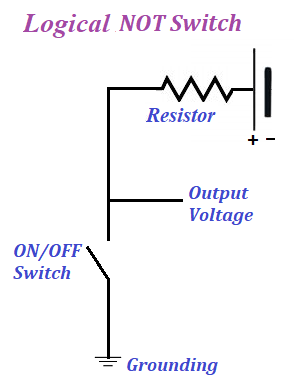When ON/OFF switch is in OFF position, there is no current in a circuit and the potential at the output point will be positive as on the corresponding battery terminal.

If we change the switch to ON position, there will be an electric current flow between the ground and the positive battery terminal, the potential at the output point will be the same as that of the grounding, that is zero.

This circuit converts ON position of the switch into zero potential at the output, while OFF position of the switch is converted into positive potential at the output.

With this in mind, all we have to change in this circuit to make it purely electronic is to implement the switch electronically.
We can use a triode for this purpose with grounded cathode.

As we know, the positive potential on the grid of a triode enhances the attraction of an anode for an electron cloud around a grounded but heated cathode, thereby opening the movement of electrons from cathode to anode. Since cathode is grounded, all electrons that moved to an anode will be compensated from the ground, and there will be a steady flow of electrons from cathode to anode, which is equivalent to a switch to be in ON position.

At the same time, grounded (zero potential) grid does not help the electrons of the grounded cathode to reach the positive anode, there will be very weak, if any, electric current between anode and cathode, which with proper parameters of the components of a circuit can be brought practically to zero, which is equivalent to a switch to be in OFF position.

This brings us to the following circuit that implements the logical NOT operation (inverter).This is a very primitive implementation, only for educational purposes. The real inverters are more complex and usually implemented using transistors, which we will discuss later in the course.

### Logical XOR: UNIZOR.COM - Physics4Teens - Electromagnetism - Electronics

Notes to a video lecture on http://www.unizor.com

Logical XOR Implementation

Let's analyze how diodes can be used to implement operation XOR (or addition by modulo 2) of mathematical (and computer) logic.

The standard is that TRUE or 1 is represented by some positive potential on a wire relative to the ground, while FALSE or 0 is represented by zero potential relative to the ground.

Exclusive OR (XOR) operation is represented by a symbol of addition in a circle .
The rules are:
0 ⊕ 0 = 0
0 ⊕ 1 = 1
1 ⊕ 0 = 1
1 ⊕ 1 = 0

Now let's design a schema that represents a logical operation XOR of two logical values.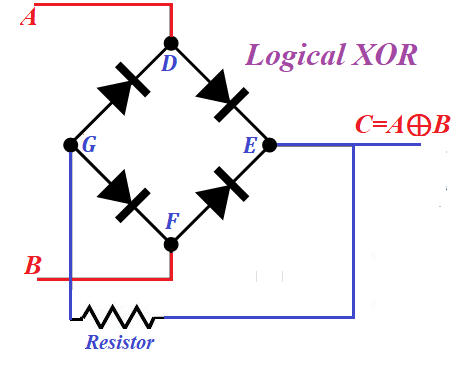There are four variations of input values A and B:
A=0, B=0
A=0, B=1
A=1, B=0
A=1, B=1

For each of these cases we will determine the output value at point C.

1. If A=0 and B=0, that is the potential is zero at both points relative to the ground and points D and F are at zero potential as well. In theory, electrons from hot cathodes in diodes at segments ED and EF can move to corresponding anodes only if these anodes are positively charged. But this is not the case since the potentials at points D and F are zero. Therefore, no electrons escape point E. Point G is connected only to anodes and, therefore, no electrons escape this point either. So, at point C the potential is zero, that is C=0

2. Consider a case of A=0 and B=1, that is the potential is zero at points A and D, but is positive (deficiency of electrons) at points B and F relative to the ground.
A diode on a segment EF has its anode positively charged from point F. Therefore, electrons in the cloud produced by thermionic emission on its cathode will be attracted to positively charged anode, thus creating a deficiency of electrons (positive potential) on this cathode and at points E and C. Point C becomes positively charged, that is C=1.
Deficiency of electrons at point E cannot be compensated through a segment DE because a diode on this segment is working in an opposite direction. So, deficiency of electrons will be compensated from point G from point G, which becomes positively charged and attracts electrons from point D through a diode at segment DG. These new electrons, coming from point A to point D, through thermionic emission to point G and to point E, will be immediately dispatched to a thermionic emission cloud and attracted by positively charged anode of a diode on EF segment, thus maintaining a constant flow of electrons from point G (neutral) to point E (positive).
This process of electrons moving from zero potential at point A to point D, to thermionic emission cloud on a cathode of DG segment, to point G, to point E will continue as long as point B has positive potential.
Therefore, the cathode of a diode on a segment EF will always be positively charged, and so is a wire at points E and C connected to it. That means that C=1.

3. If A=1 (positively charged, that is deficiency of electrons) and B=0 (neutral), situation is analogous to a previous one, except all the electrons will flow from point B to point F, to cathode of a diode on a segment FG to point G, to point E, to thermionic cloud of a cathode on segment ED to positively charged points D and A. Since there is a deficiency of electrons at point E, the potential at point C will be positive, that is C=1.

4. Case A=1 and B=1 (both deficient of electrons). There is no source of electrons to compensate this deficiency. If point E was connected to such a source, there would be a flow of electrons from it through segments ED and EF, but this is not the case. No electrons are moving through point G either. Therefore, potential at points E, G and C is zero, that is C=0

As we see, if any one input potentials at points A and B equal to 1, the output C=1 as well. If both A=0 and B=0, the potential at C is zero, that is C=0. If both A=1 and B=1, the potential at C is also zero, that is C=0.

That fully corresponds to the rules of logical XOR operation.

Therefore, the circuit above is a proper electronic implementation of logical operation of exclusive OR (or addition by modulo 2).

Obviously, the real implementation requires some concrete values for voltage, resistance, characteristics of diodes etc. that we will not address here, as our purpose is to introduce only the principle behind this implementation.

### Logical AND: UNIZOR.COM - Physics4Teens - Electromagnetism - Electronics

Notes to a video lecture on http://www.unizor.com

Logical AND Implementation

Let's analyze how diodes can be used to implement operation AND of mathematical (and computer) logic.

The standard is that TRUE or 1 is represented by some positive potential on a wire relative to ground, while FALSE or 0 is represented by zero potential relative to ground.

Conjunction AND is represented by a symbol of multiplication · or an ampersand &.
The rules are:
0 & 0 = 0
0 & 1 = 0
1 & 0 = 0
1 & 1 = 1

Now let's design a schema that represents a logical operation AND of two logical values.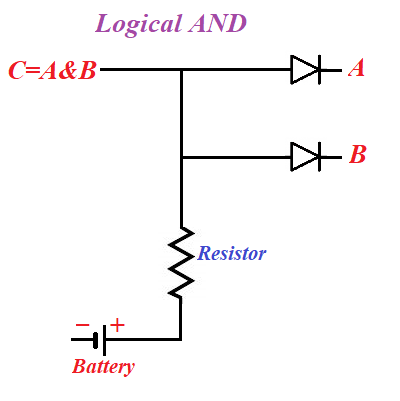Here points A and B are on the cathode sides of corresponding diodes, while both anodes are connected to a positive potential wire from a battery.

If both A and B represent the value of 1 (that is, have positive potential and deficiency of electrons), there is no difference in potentials between anodes and corresponding cathodes, no thermionic clouds and the potential at point C will be positive, that is C=1 in this case.

If the potential at point A or at point B, or at both of these points is maintained at 0, electrons from thermionic cloud will fly to a corresponding anode and, further, neutralize any positive potential (deficiency of electrons) that might exist at point C.
Therefore, in this case the logical value at point C will be equal to 0.

This is exactly the same as the definition of logical operation AND between two boolean variables A and B.

Therefore, the circuit above is a proper electronic implementation of logical operation of conjunction.

Obviously, the real implementation requires some concrete values for voltage, resistance, characteristics of diodes etc. that we will not address here, as our purpose is to introduce only the principle behind this implementation.

### Logical OR: UNIZOR.COM - Physics4Teens - Electromagnetism - Electronics

Notes to a video lecture on http://www.unizor.com

Logical OR Implementation

Let's analyze how diodes can be used to implement operation OR of mathematical (and computer) logic.

The standard is that TRUE or 1 is represented by some positive potential on a wire relative to the ground, while FALSE or 0 is represented by zero potential relative to the ground.

Disjunction OR is represented by a symbol of addition + or a vertical bar |.
The rules are:
0 | 0 = 0
0 | 1 = 1
1 | 0 = 1
1 | 1 = 1

Now let's design a schema that represents a logical operation OR of two logical values.

Assume, the wires A and B, connected to corresponding anodes of two diodes, represent some logical values, TRUTH (1) or FALSE (0) and find the value represented by wire C on the following pictureThere are four variations of values A and B:
A=0, B=0
A=0, B=1
A=1, B=0
A=1, B=1

For each of these cases we will determine the value at point C.

1. If A=0 and B=0, that is the potential is zero at both points relative to ground, there is no electric current anywhere. Even with cathode on any of the diodes heated, there is no current since there is no positive charge on anode to attract the electrons in the cloud produced by thermionic emission. So, at point C the potential is zero, that is C=0

2. Consider a case of A=0 and B=1, that is the potential is zero at point A, but is positive at point B relative to the ground.
The electrons in the cloud produced by thermionic emission on cathode opposite to point B will be attracted to positively charged anode at point B, thus creating a deficiency of electrons (positive potential) on this cathode.
New electrons from the neutral ground will compensate the loss of electrons on this positively charged cathode, but will be immediately dispatched to a thermionic emission cloud and attracted by positively charged anode at point B, thus maintaining a constant flow of electrons from the neutral ground through positively charged cathode to point B.
This process of electrons moving from the ground to positively charged cathode opposite to point B, to thermionic emission cloud and to point B will continue as long as point B has positive potential.
Therefore, the cathode opposite to point B will always be positively charged, and so is a wire at point C connected to it. That means that C=1.

3. If A=1 and B=0, situation is analogous to a previous one, except all the electrons will flow to point A. The potential at point C will be positive, that is C=1.

4. Case A=1 and B=1 is not much different. Now the electrons will flow from the ground to both points A and B. The point C will be positively charged, that is C=1.

As we see, if any one or both input potentials at points A and B equal to 1, the output C=1 as well. Only if both A=0 and B=0, the potential a C is zero, that is C=0.

That fully corresponds to the rules of logical OR operation.

Therefore, the circuit above is a proper electronic implementation of logical operation of disjunction.

Obviously, the real implementation requires some concrete values for voltage, resistance, characteristics of diodes etc. that we will not address here, as our purpose is to introduce only the principle behind this implementation.

## Friday, March 5, 2021

### Electronic Logic: UNIZOR.COM - Physics4Teens - Electromagnetism - Electr...

Notes to a video lecture on http://www.unizor.com

Electronic Logic

Let's analyze how diodes can be used to implement operations of mathematical (and computer) logic.
These are operations on variables that can have only two possible values - TRUE (in many cases represented by number 1) and FALSE (represented by 0).

TRUE and FALSE in Electronics

First of all, we have to agree on what represents logical TRUE and FALSE or 1 and 0 in electrical circuits.
The standard is that TRUE or 1 is represented by some positive potential on a wire relative to ground, while FALSE or 0 is represented by zero potential relative to ground.

Consider the following circuit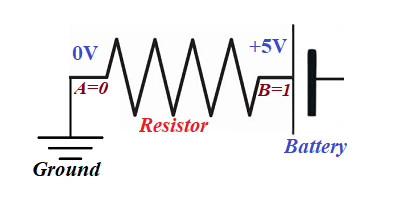According to our rules about what represents logical TRUE (1) and FALSE (0), the logical value at point A is FALSE (0) and at point B it is TRUE (1).

Binary logical operations

Disjunction OR (sometimes represented by symbol of addition + or vertical bar |).
The rules are: 0|0=0, 0|1=1, 1|0=1, 1|1=1

Conjunction AND (might be represented by symbol of multiplication · or ampersand &),
The rules are: 0&0=0, 0&1=0, 1&0=0, 1&1=1

Exclusive disjunction XOR (might be represented by symbol ).
The rules are: 00=0, 01=1, 10=1, 11=0

Unary logical operation

Negation NOT (sometimes represented by exclamation sign ! or symbol ¬).
The rules are: ¬0=1, ¬1=0

Implementation

Implementation of binary logical operations in electronics involves developing a schema with two input contacts, A and B, and one output contact C. On two input contacts there might be four different combinations of electric potential:
A=0, B=0
A=0, B=1
A=1, B=0
A=1, B=1
For each of these combinations the value of electric potential at output point C should correspond to the rules of the corresponding binary logical operation implemented in this schema.

Implementation of unary logical operations in electronics involves developing a schema with one input contacts A and one output contact B. On input contact A there might be two different electric potentials:
A=0
A=1
For each of these combinations the value of electric potential at output point B should correspond to the rules of the corresponding unary logical operation implemented in this schema.

Examples of implementation of certain logical operations in electronics are presented in subsequent lectures.

## Tuesday, March 2, 2021

### Electronics - Triode: UNIZOR.COM - Physics4Teens - Electromagnetism - Usage

Notes to a video lecture on http://www.unizor.com

Electronics: Triode

Triodes are another class of relatively simple electronic components that are present in practically all electronic devices.
The main purpose of a triode is to amplify the electric current in addition to rectifying it.

Placing a negatively charged grid between a source of an electron cloud (cathode) and a plate receiving electrons produced by this thermionic emission (anode) allows to regulate the flow of electrons from cathode to anode.

The greater negative charge of an electrostatic field of a grid is - the more electrons will be repelled back to cathode and will not reach anode.
The electrically neutral grid causes a triode to act as a diode since the grid is no longer repels electrons, so they are attracted and fly to anode without any resistance.

There is a direct correlation between the voltage of a grid relatively to cathode and the amperage of the current reaching the anode.

Below is a schematic representation of a vacuum tube triode with a heated filament separated from a cathode, so the battery which heats the filament does not interfere with current from cathode to anode.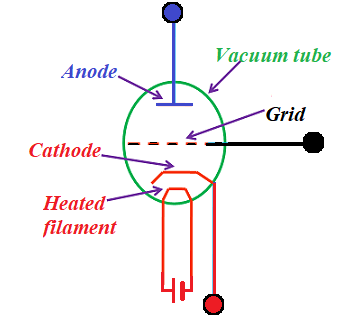The following schema represents the basic principle of amplifying a signal using a triode.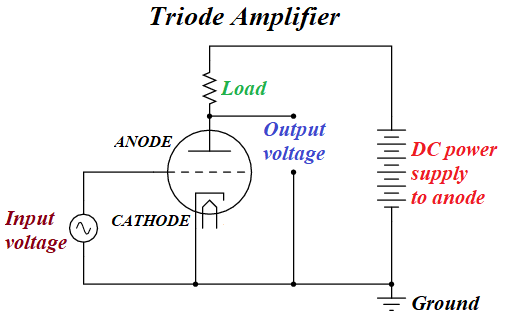The DC power supply causes a flow of electrons from an electron cloud around cathode, caused by thermionic emission, to anode plate.
Input voltage is applied to a grid inside a triode, that affects the flow of electrons from cathode to anode.

Varying the base voltage of DC power supply, we can regulate the strength of the output voltage relatively to input voltage.
Output voltage changes synchronously with the input voltage with the same frequency but different amplitude. This enables amplifying the input signal.

Vacuum tube triodes are rarely used nowadays. Semiconductors and integrated circuits are the main technological base. Basic functionality of triodes is implemented in semiconductors called transistors. These will be discussed in a separate chapter of this course dedicated to semiconductors.